# 频率域波图像增强

Posted by jjx on December 25, 2016

• 频率域图像增强
• 高通滤波器和低通滤波器
• 本章的典型案例分析
• 利用频域滤波消除周期噪声

### 频域滤波基础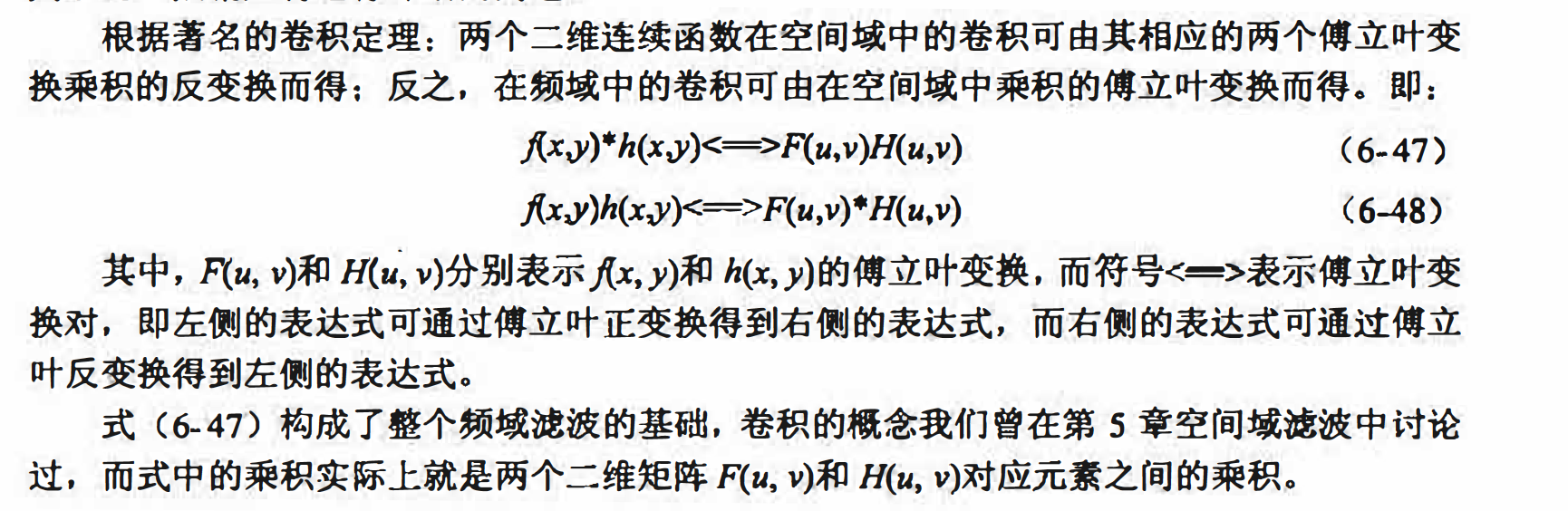(1）计算原始图像f(x,y） 的DFT， 得到F(u, v).
(2）将频谱F(u,v）的零频点移动到频谱图的中心位置。
(3）计算滤波器函数H(u, v）与F(u,v）的乘积G(u, v).
(4）将频谱G(u, v）的零频点移回到频谱图的左上角位置。
(5）计算第（4）步计算结果的傅立叶反变换g(x,y） 。
(6）取g(x,y ）的实部作为最终滤波后的结果图像．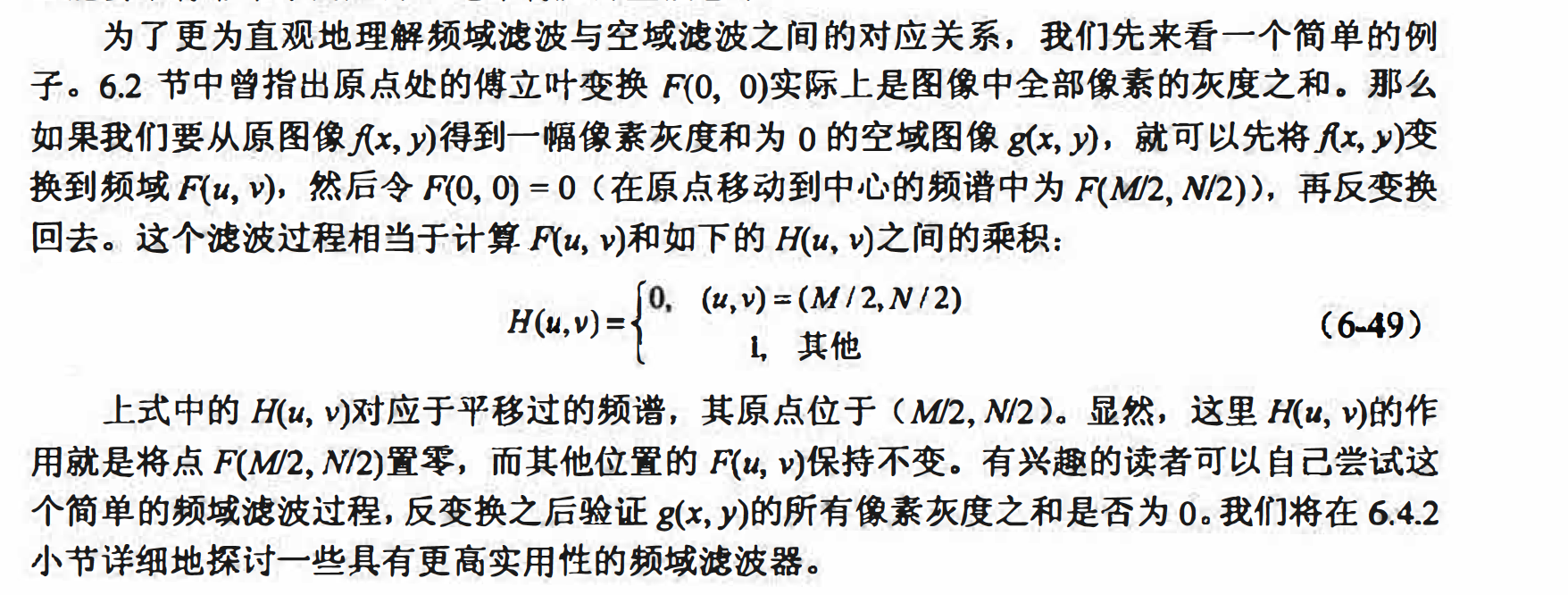matlab实现

function out = imfreqfilt(I, ff)
% imfreqfilt函数			对灰度图像进行频域滤波
% 参数I				输入的空域图像
% 参数ff				应用的与原图像等大的频域滤镜

if (ndims(I)==3) && (size(I,3)==3)   % RGB图像
I = rgb2gray(I);
end

if (size(I) ~= size(ff))
msg1 = sprintf('%s: 滤镜与原图像不等大，检查输入', mfilename);
msg2 = sprintf('%s: 滤波操作已经取消', mfilename);
eid = sprintf('Images:%s:ImageSizeNotEqual',mfilename);
error(eid,'%s %s',msg1,msg2);
end

% 快速傅立叶变换
f = fft2(I);

% 移动原点
s = fftshift(f);

% 应用滤镜及反变换
out = s .* ff; %对应元素相乘实现频域滤波
out = ifftshift(out);
out = ifft2(out);

% 求模值
out = abs(out);

% 归一化以便显示
out = out/max(out(:));


#### 频域低通滤波器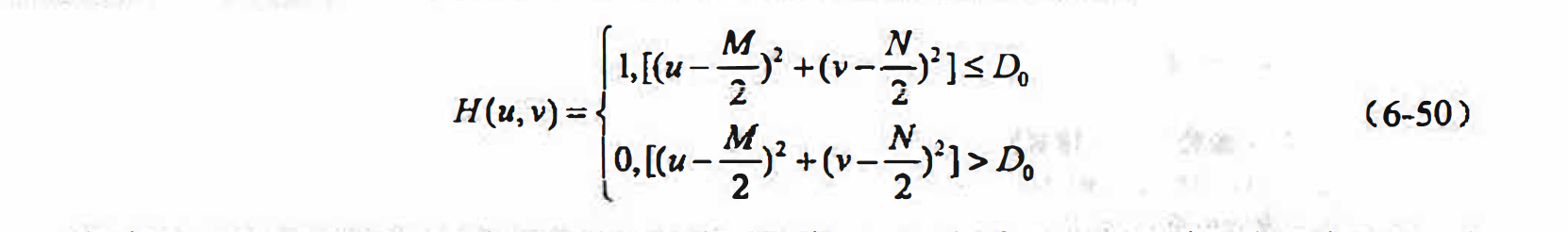matlab实现

function out = imidealflpf(I, freq)
% imidealflpf函数			构造理想的频域低通滤波器
% I参数				输入的灰度图像
% freq参数				低通滤波器的截止频率
% 返回值：out – 指定的理想低通滤波器
[M,N] = size(I);
out = ones(M,N);
for i=1:M
for j=1:N
if (sqrt(((i-M/2)^2+(j-N/2)^2))>freq)
out(i,j)=0;
end
end
end

ff = imidealflpf(I,20);
out = imfreqfilt(I,ff);

figure(1);
subplot(2,2,1);
imshow(I);
title('Source');

temp = fft2(I);
temp = fftshift(temp);
temp = log(1+abs(temp));
figure(2);
subplot(2,2,1);
imshow(temp,[]);
title('Source');

figure(1);
subplot(2,2,2);
imshow(out);
title('Ideal LPF,frq = 20');

temp = fft2(out);
temp = fftshift(temp);
temp = log(1+abs(temp));
figure(2);
subplot(2,2,2);
imshow(temp,[]);
title('Ideal LPF,frq = 20');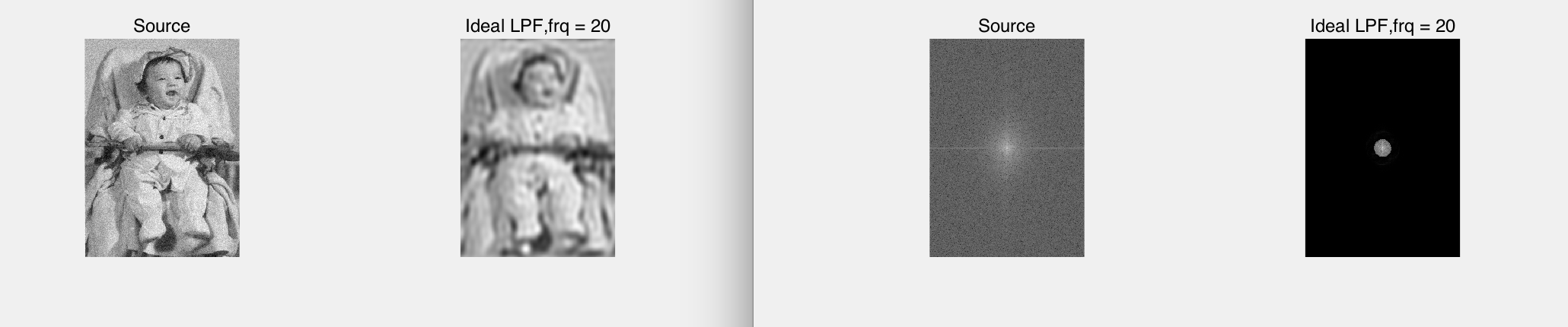由图可知，当截止频率非常低时， 只有非常靠近原点的低频成份能够通过， 图像模糊严重：截止频率越高， 通过的频率成份就越多， 图像模糊的程度越小， 所获得的图像也就越接近原图像。 但可以看出， 理想低通滤波器并不能很好地兼顾噪声滤除与细节保留两个方面， 这和空域中采用平均模板时的情形比较类似。 下面将介绍频域的高斯低通滤 波器并比较它与理想低通滤波器的处理效果。

#### 高斯低通滤波器及其实现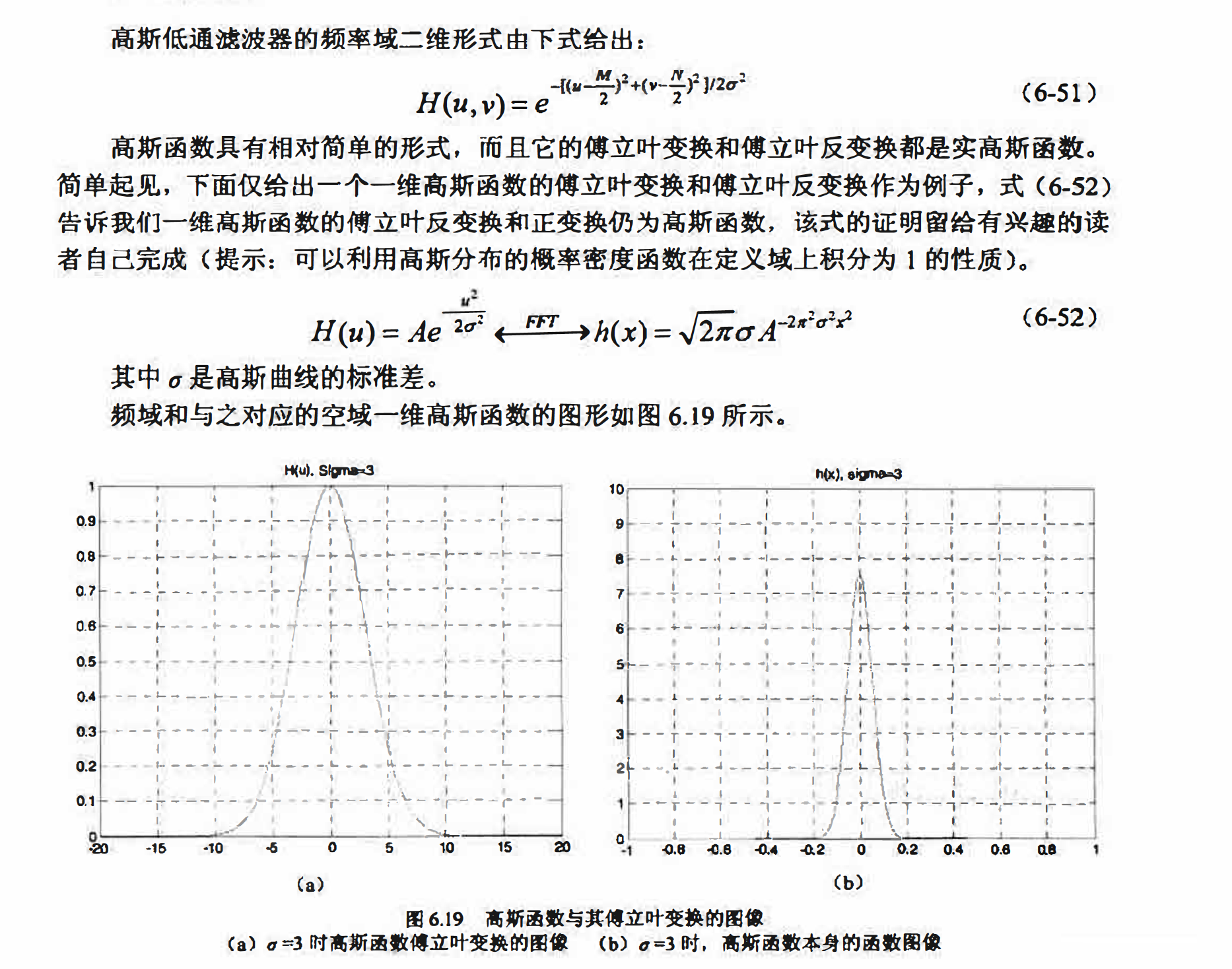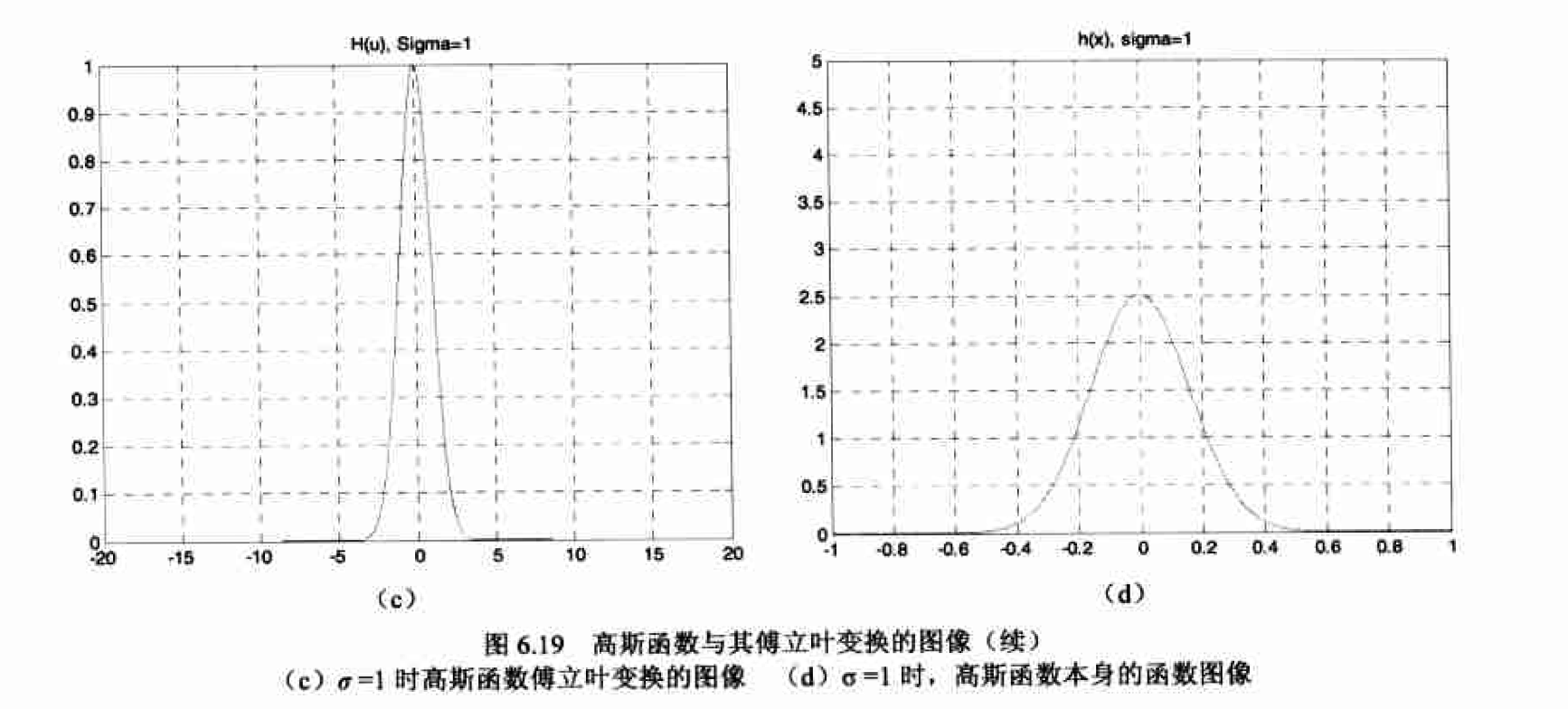matlab实现

function out = imgaussflpf(I, sigma)
% imgaussflpf函数     		构造频域高斯低通滤波器
% I参数				输入的灰度图像
% sigma参数			高斯函数的Sigma参数

[M,N] = size(I);
out = ones(M,N);
for i=1:M
for j=1:N
out(i,j) = exp(-((i-M/2)^2+(j-N/2)^2)/2/sigma^2);
end
end

ff = imgaussflpf(I,20);
out = imfreqfilt(I,ff);

figure(1);
subplot(2,2,1);
imshow(I);
title('Source');

temp = fft2(I);
temp = fftshift(temp);
temp = log(1+abs(temp));
figure(2);
subplot(2,2,1);
imshow(temp,[]);
title('Source');

figure(1);
subplot(2,2,2);
imshow(out);
title('Gauss LPF,sigma=20');

temp = fft2(out);
temp = fftshift(temp);
temp = log(1+abs(temp));
figure(2);
subplot(2,2,2);
imshow(temp,[]);
title('Gauss LPF,sigma=20');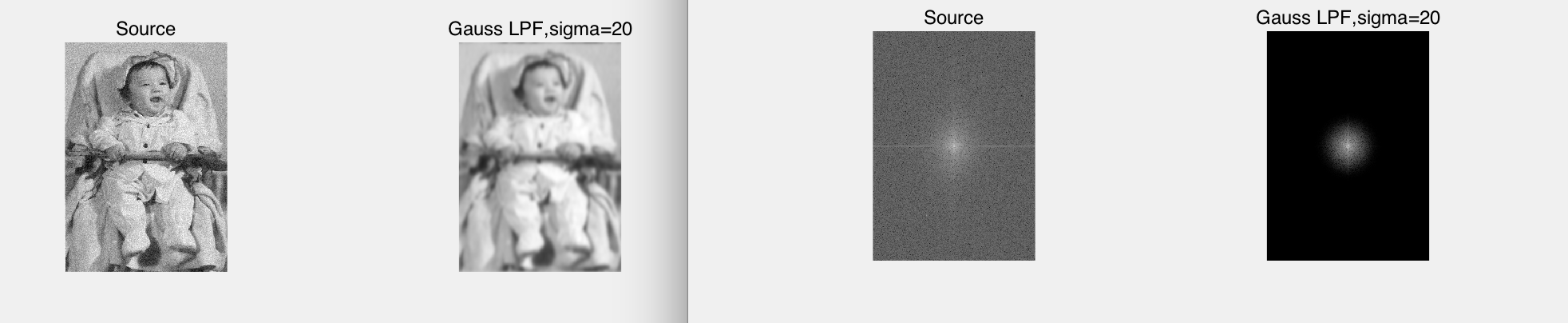#### 频率域高通滤波器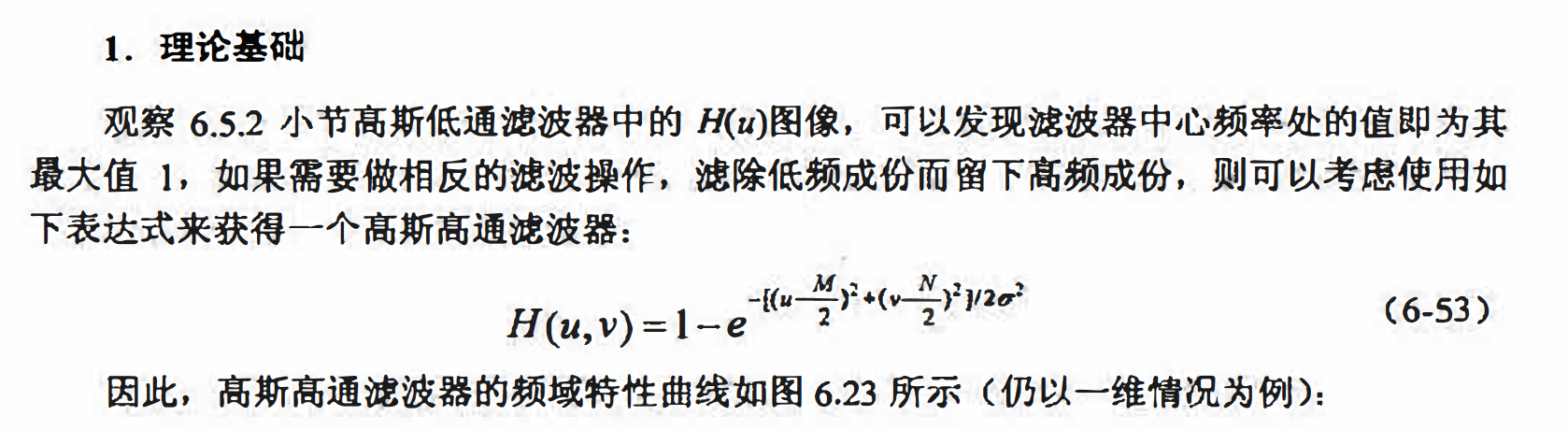matlab实现

function out = imgaussfhpf(I, sigma)
% imgaussfhpf函数         构造频域高斯高通滤波器
% I参数               输入的灰度图像
% sigma参数           高斯函数的Sigma参数

[M,N] = size(I);
out = ones(M,N);
for i=1:M
for j=1:N
out(i,j) = 1 - exp(-((i-M/2)^2+(j-N/2)^2)/2/sigma^2);
end
end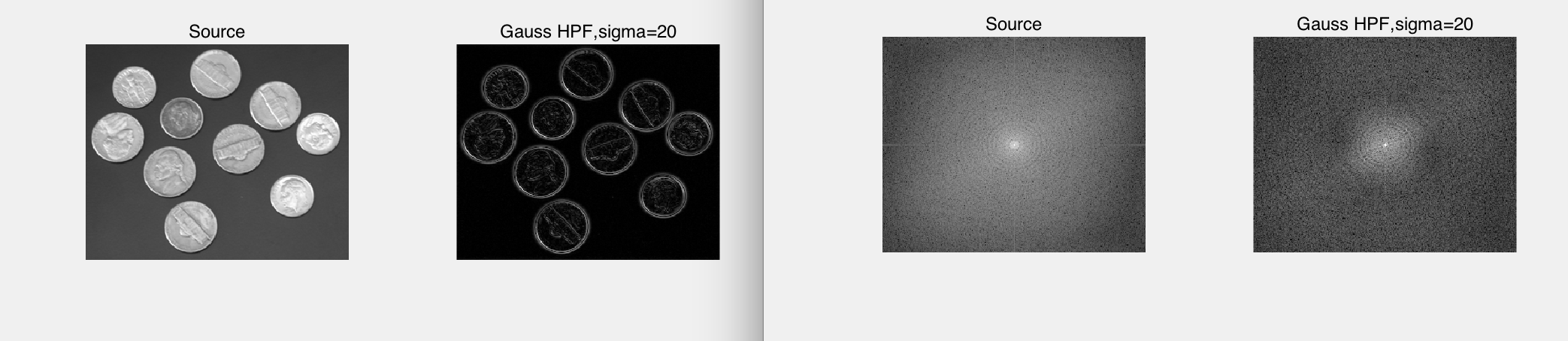高斯高通滤波器可以较好地提取图像中的边缘信息， Sigma参数取值越小， 边缘提取越 不精确， 会包含更多的非边缘信息： Sigmaa 参数取值越大， 边缘提取越精确， 但可能包含不完整的边缘信息。

matlab实现

function out = imlapf(I)
% imlapff函数         构造频域拉普拉斯滤波器
% I参数               输入的灰度图像

[M,N] = size(I);
out = ones(M,N);
for i=1:M
for j=1:N
out(i,j) = -((i-M/2)^2+(j-N/2)^2);
end
end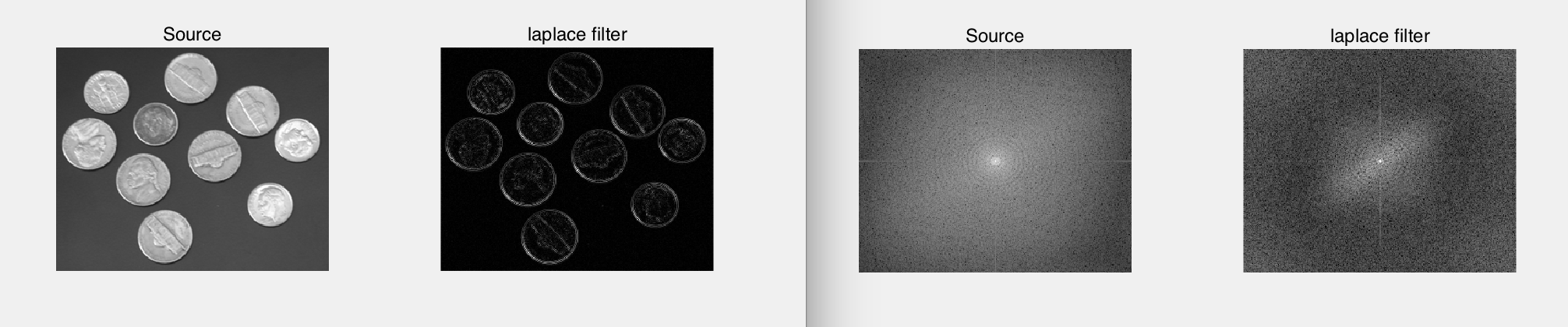#### Matlab 综合案例一一利用频域滤波消除周期噪声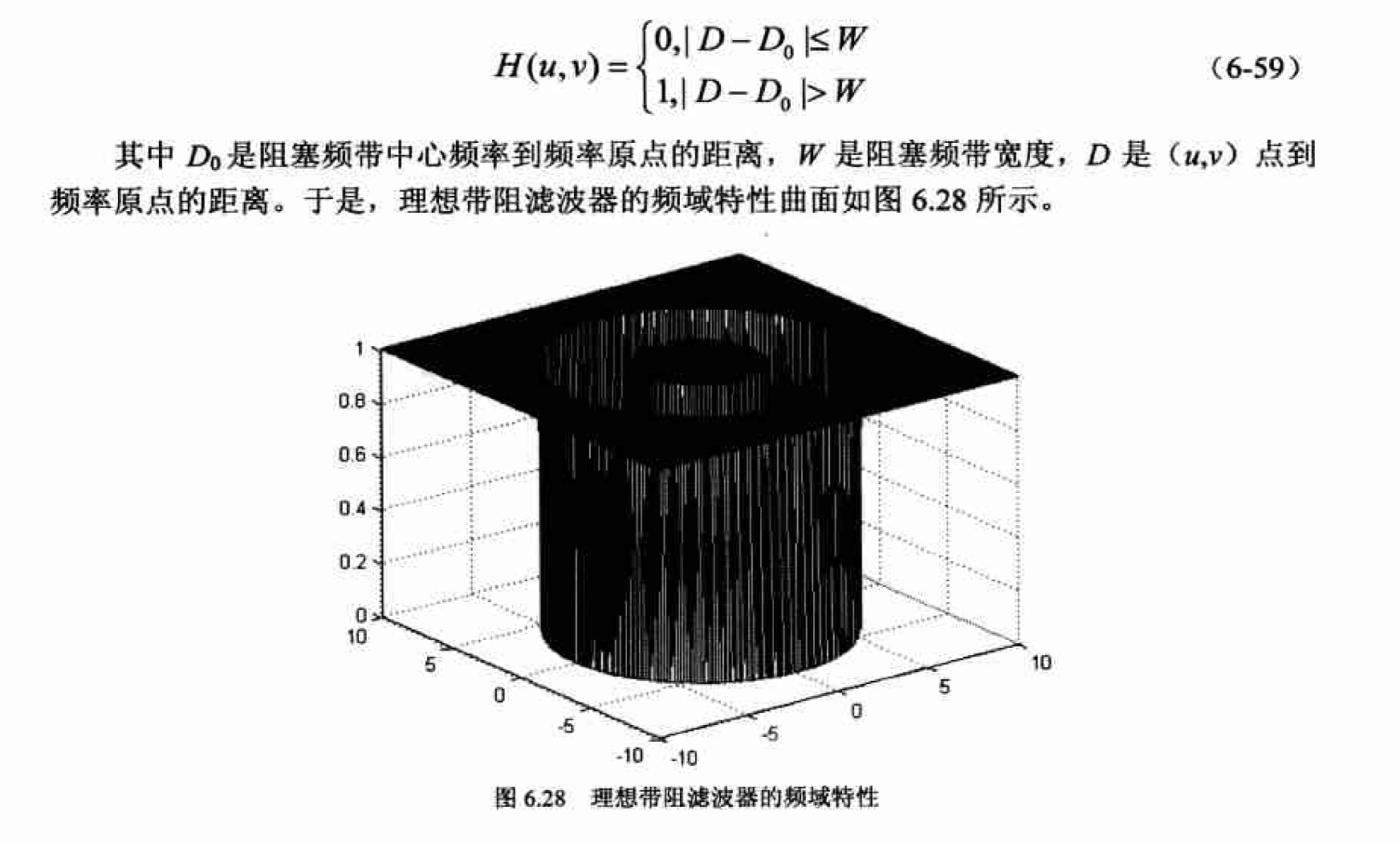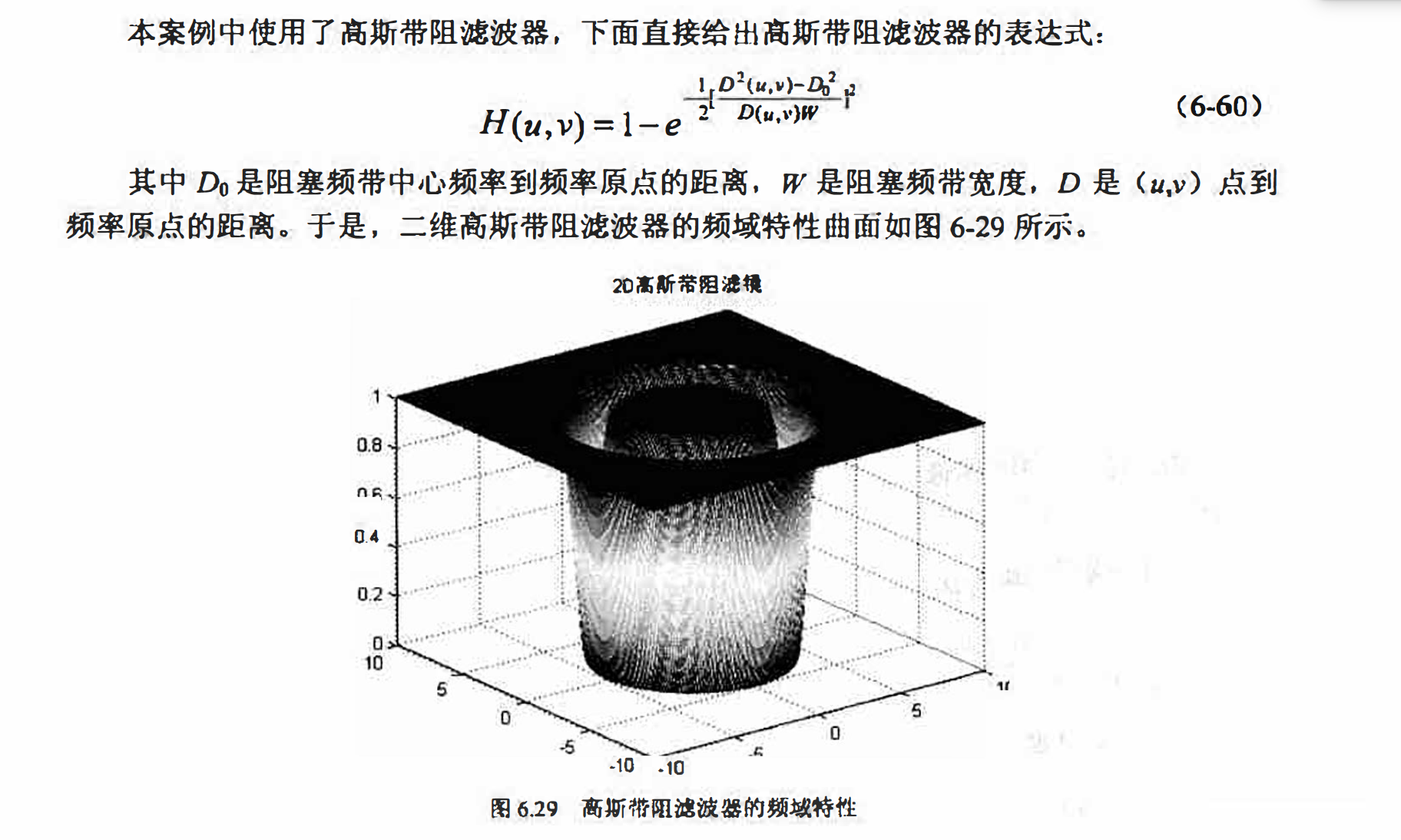matlab实现

O = imread('pout.tif');
[M,N] = size(O);
I = O;
for i = 1:M
for j = 1:N
I(i,j) = I(i,j)+20*sin(20*i)+20*sin(20*j);
end
end

subplot(1,2,1);
imshow(O);
title('Source');

subplot(1,2,2);
imshow(I);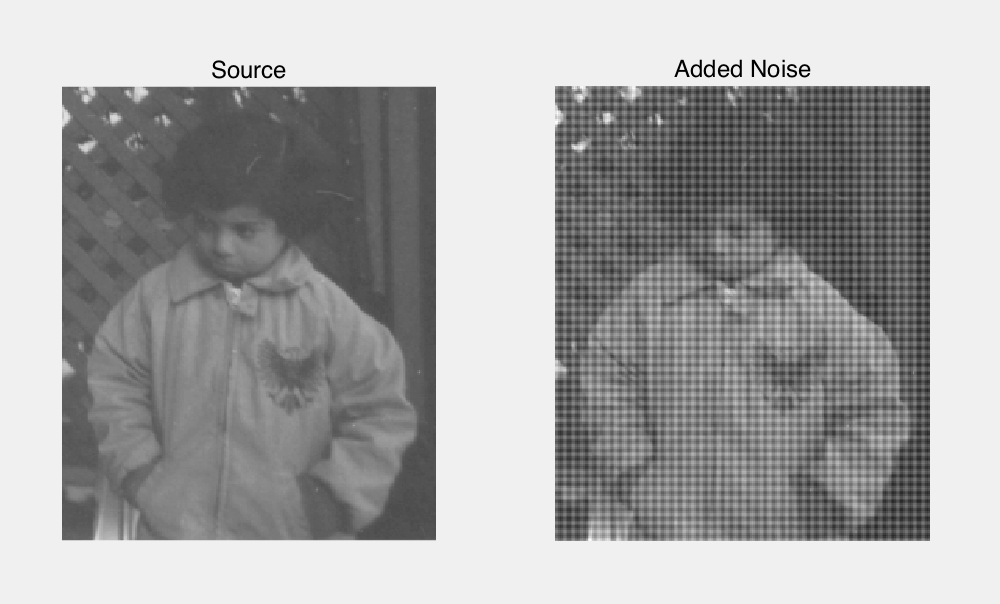i_f = fft2(I);
i_f = fftshift(i_f);
i_f = abs(i_f);
i_f = log(1+i_f);

o_f = fft2(O);
o_f = fftshift(o_f);
o_f = abs(o_f);
o_f = log(1+o_f);

figure(1);
subplot(1,2,1);
imshow(o_f,[]);
title('Source');

subplot(1,2,2);
imshow(i_f,[]);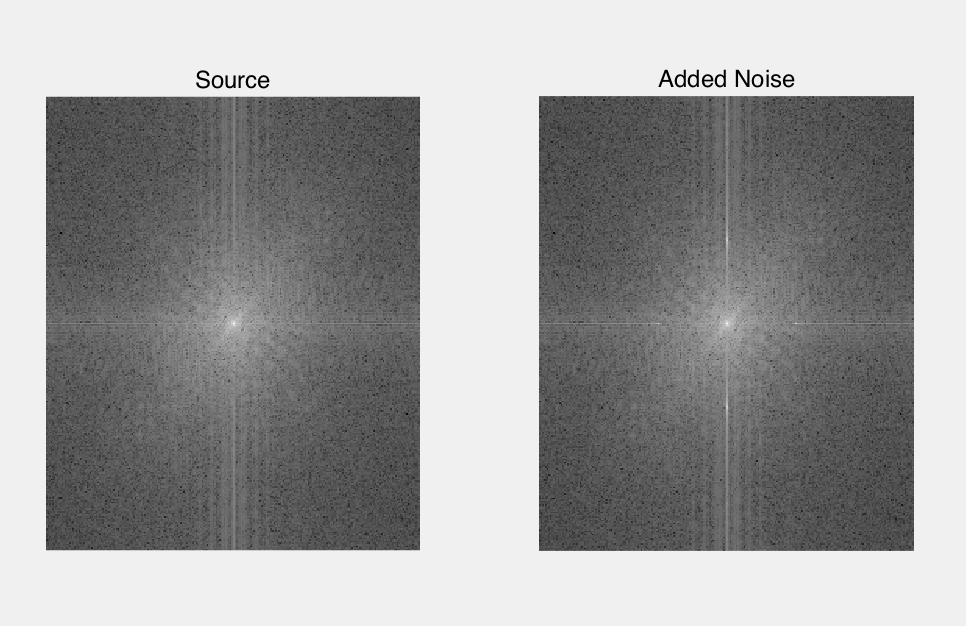ff = imgaussfbrf(I,50,5);
figure(1);
subplot(2,2,1);
imshow(ff,[]);

out = imfreqfilt(I,ff);
temp = fft2(out);
temp = fftshift(temp);
temp = log(1+abs(temp));
subplot(2,2,2);
imshow(temp,[]);

subplot(2,2,3);
imshow(I);
title('Source');

subplot(2,2,4);
imshow(out);
title('Gauss Filter');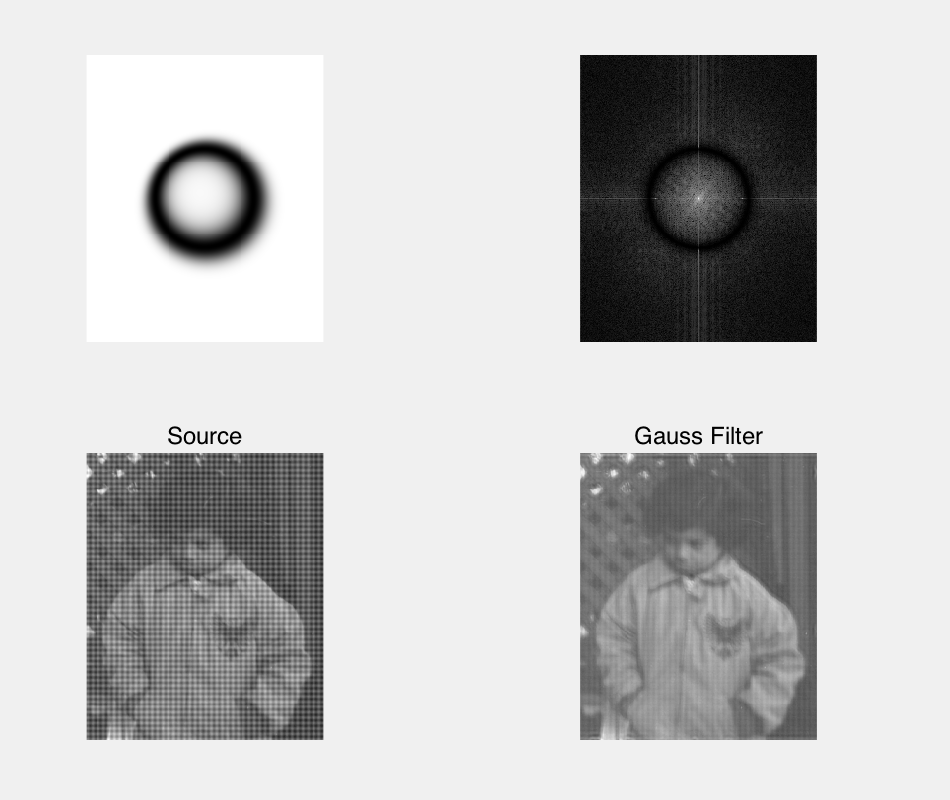上述程序运行后得到的高斯带阻滤波器的最终滤波效果如图所示。我们看到周期噪声被很好地消除， 这样的效果在空域中是很难实现的。